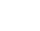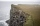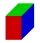# The spacecraft

The spacecraft spotted a radar device at altitude angle alpha = 34 degrees 37 minutes and had a distance of u = 615km from Earth's observation point.
Calculate the distance d of the spacecraft from Earth at the moment of observation. Earth is considered a sphere with a radius of 6378km.

Correct result:

x =  368.383 km

#### Solution:

$α=34 +\dfrac{ 37 }{ 60 }=\dfrac{ 2077 }{ 60 } \doteq 34.6167 \ ^\circ \ \\ \ \\ u=615 \ \text{km} \ \\ R=6378 \ \text{km} \ \\ β=90 + α=90 + 34.6167 \doteq \dfrac{ 7477 }{ 60 } \doteq 124.6167 \ ^\circ \ \\ \ \\ (x+R)^2=u^2 + R^2 - 2 \cdot \ u \cdot \ R \cdot \ \cos α \ \\ \ \\ x=\sqrt{ u^2 + R^2 - 2 \cdot \ u \cdot \ R \cdot \ \cos β ^\circ } - R=\sqrt{ u^2 + R^2 - 2 \cdot \ u \cdot \ R \cdot \ \cos 124.61666666667^\circ \ } - R=\sqrt{ 615^2 + 6378^2 - 2 \cdot \ 615 \cdot \ 6378 \cdot \ \cos 124.61666666667^\circ \ } - 6378=\sqrt{ 615^2 + 6378^2 - 2 \cdot \ 615 \cdot \ 6378 \cdot \ (-0.568083) } - 6378=368.383 \ \text{km}$We would be very happy if you find an error in the example, spelling mistakes, or inaccuracies, and please send it to us. We thank you!Tips to related online calculators
Cosine rule uses trigonometric SAS triangle calculator.

#### You need to know the following knowledge to solve this word math problem:

We encourage you to watch this tutorial video on this math problem:

## Next similar math problems:The regular quadrilateral pyramid has a base edge a = 1.56 dm and a height h = 2.05 dm. Calculate: a) the deviation angle of the sidewall plane from the base plane b) deviation angle of the side edge from the plane of the base
• Right triangleA right triangle ABC is given, c is a hypotenuse. Find the length of the sides a, b, the angle beta if c = 5 and angle alfa = A = 35 degrees.
• Five circlesOn the line segment CD = 6 there are 5 circles with radius one at regular intervals. Find the lengths of the lines AD, AF, AG, BD, and CE
• Digging a pitThe pit has the shape of a regular quadrilateral truncated pyramid. The edges of the bases are 14m and 10m long. The sidewalls form an angle of 135° with a smaller base. Determine how many m3 of soil were excavated when digging the pit?
• Maximum of volumeThe shell of the cone is formed by winding a circular section with a radius of 1. For what central angle of a given circular section will the volume of the resulting cone be maximum?The height of a regular quadrilateral prism is v = 10 cm, the deviation of the body diagonal from the base is 60°. Determine the length of the base edges, the surface, and the volume of the prism.
• Rhombus diagonalsIn the rhombus ABCD are given the sizes of diagonals e = 24 cm; f = 10 cm. Calculate the side length of the diamond and the size of the angles, calculate the content of the diamond
• Find theFind the surface area of a regular quadrilateral pyramid which has a volume of 24 dm3 and a height of 45 cm.
• A cliffA line from the top of a cliff to the ground passes just over the top of a pole 5 ft high and meets the ground at a point 8 ft from the base of the pole. If the point is 93 ft from the base of the​ cliff, how high is the​ cliff?
• Isosceles triangleCalculate the area of an isosceles triangle, the base of which measures 16 cm and the arms 10 cm.
• Surface of the coneCalculate the surface of the cone if its height is 8 cm and the volume is 301.44 cm3.
• CalculateCalculate the surface and volume of the cone that results from the rotation of the right triangle ABC with the squares 6 cm and 9 cm long around the shorter squeegee.
• 9-gon pyramidCalculate the volume and the surface of a nine-sided pyramid, the base of which can be inscribed with a circle with radius ρ = 7.2 cm and whose side edge s = 10.9 cm.
• How manyHow many m2 of copper sheet is needed to replace the roof of a conical tower with a diameter of 13 meters and a height of 24 meters, if we count 8% of the material for bending and waste?
• Surface and volumeFind the surface and volume of the rotating cone if the circumference of its base is 62.8 m and the side is 25 m long.
• Sphere parts, segmentA sphere with a diameter of 20.6 cm, the cut is a circle with a diameter of 16.2 cm. .What are the volume of the segment and the surface of the segment?
• Body diagonalFind the length of the body diagonal of a cuboid with lengths of 16 cm, 7 cm, and 4 cm.